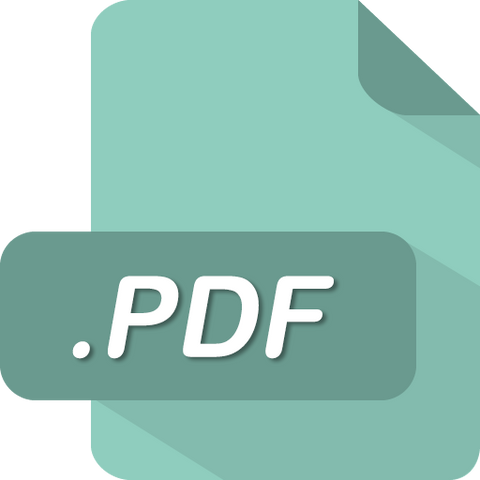# FINC 331 FINC331 Quiz 2 Answers (UMUC)

soffix

• \$12.99

FINC 331 Quiz 2

1. You purchase a two year annuity for \$2800. The annuity pays \$1500 each year. What is the annuity's approximate IRR?
2. You can purchase two three-year annuities today. One is valued at \$2000, the other at \$4000. The 1st annuity begins paying \$1000 in a year. The 2nd annuity begins paying \$1500 in two years. The interest rate is 5%. What is the PV of the portfolio? (Assume anything not paid during the 3 years will be paid to you at the end of the 3 years.)
3. Of the following car financing options, which one would you prefer while assuming that you prefer paying the least amount of dollars and that you face a 10% annual compound interest rate on all your financial decisions?
4. Which of the following could be an appropriate period used in a present value calculation?
5. In a year, you expect to receive a payment of \$1 million in a year. That annual interest rate is 5%. What is the present value of the future payment?
6. Assume you invest money in a bond that will pay you \$250,000 in four years. The bond has an annual interest rate of 5%. You do not receive interest payments while you own the bond; it is zerocoupon. What is the bond's present value?
7. What is the present value of \$100,000 that will be received 5 years from today if you face a 10% compound interest rate every year (rounded up to the nearest dollar)?
8. Which of the following is the definition of the Macaulay duration?
9. Given an inflation rate of 3% and a real rate of 5%, what is the corresponding nominal rate?
10. A bond has a coupon rate of 7% and a yield to maturity rate of 8%. The bond is ____.
11. A bond grants its holder the option to sell the bond back to the issuer at a fixed price at a fixed date prior to the bond's maturity. When evaluating the bond's value, the company should calculate the bond's _____.
12. Which of the following statements regarding bonds and par values is true?
13. A zero-coupon bond has a face value of \$1000 and a market value of \$800. The bond will mature in 5 years. What is its yield to maturity?
14. An annuity has an interest rate of 7% and makes a quarterly payment of \$2000. The annuity is to last for 5 years. What is the present value of the annuity.
15. Which of the following influences a bond's price risk?

Sale

Unavailable

Sold Out